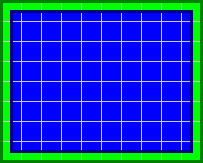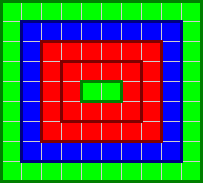### Problem Statement

A sequence of rectangles is drawn on a plane that is initially completely white. Each rectangle lies strictly inside the previous one. Each rectangle is completely colored in one of three colors - red, green or blue. The colors do not mix, so when a rectangle is drawn inside another rectangle, the new color replaces any existing color in that same area.

The rectangles are described by the int[]s width and height, and the String color. The width and height of the i-th drawn rectangle are width[i] and height[i], respectively, and its color is the i-th character of color. The characters 'R', 'G' and 'B' represent red, green and blue, respectively.

After all the rectangles are drawn, calculate the total area occupied by red, the total area occupied by green, and the total area occupied by blue. Return the maximum of these three areas.

### Definition

 Class: ChainOfRectangles Method: getMaximalArea Parameters: int[], int[], String Returns: int Method signature: int getMaximalArea(int[] width, int[] height, String color) (be sure your method is public)

### Notes

-You won't be given the information about the exact location of each rectangle, since it's not needed to solve the problem.

### Constraints

-width will contain between 1 and 50 elements, inclusive.
-height will contain the same number of elements as width.
-color will contain the same number of characters as the number of elements in width.
-Each element of width and height will be between 1 and 10000, inclusive.
-Elements of width and height will be in strictly descending order.
-Each character of color will be 'R', 'G' or 'B'.

### Examples

0)

 `{10000}` `{10000}` `"R"`
`Returns: 100000000`
 There is only one 10,000x10,000 rectangle. It's colored red, so the area covered by red is 10,000 * 10,000 = 100,000,000. The areas covered by the other two colors are 0.
1)

 `{10, 5}` `{10, 5}` `"GB"`
`Returns: 75`
 Here, the area covered by blue is 5 * 5 = 25 and the area, covered by green is 10 * 10 - 5 * 5 = 75. We return max{75, 25} = 75.2)

 `{10, 9}` `{8, 7}` `"GB"`
`Returns: 63`
 Now, the area covered by green is 17, and the area covered by blue is 63.3)

 `{10, 8, 6, 4, 2}` `{9, 7, 5, 3, 1}` `"GBRRG"`
`Returns: 36`#### Problem url:

http://www.topcoder.com/stat?c=problem_statement&pm=8192

#### Problem stats url:

http://www.topcoder.com/tc?module=ProblemDetail&rd=10785&pm=8192

ivan_metelsky

#### Testers:

PabloGilberto , Yarin , Olexiy

Simple Math